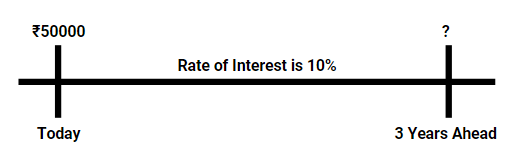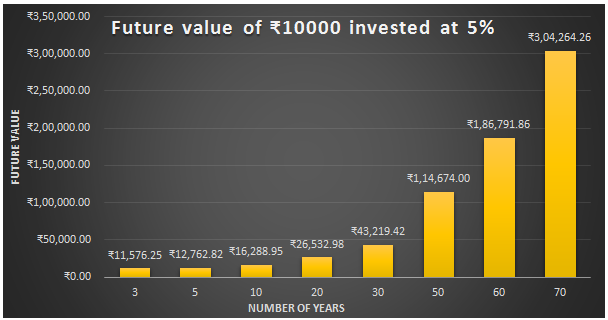# Future Value of a Single Cash Flow

• The future value of a single cash flow implies that how much will be worth of money after a few years if it is invested today at a prevalent rate of interest. E.g.: What will be the return on the maturity of a bank FD of Rs 10000 done today at a fixed rate of interest for 2 years.

### Calculation of Future Value

Example 1: Aman has invested Rs 50000 in Fixed Deposit for 3 years, with an expected return of 10% per year, he wants to know the maturity value or the future value of his investment after 3 years?
• The problem statement above is a very common doubt, almost everyone who wants to invest has this question in mind that what will be the Future Value of my Current Investment.• Lets us see how it is calculated,
• To Answer to the above question, we have a formula:
• FV = PV (1+R)T
• Where FV is the Future Value we want to calculate
• P is the Principal Invested
• R is the Expected Rate of return
• T is the time for which investment is done
• Substituting the values in the formula,
• FV = 50000 (1 + 0.1)3  = 100000 (1.1)3= Rs 66550.00
• The Formula above is the same as Formula for Calculation of compound Interest and it can be easily used to find Future Value of a sum invested today at a certain rate of interest.

Example 2: What will be Future Value of Rs 25000 invested after 10 years, if the Rate of Interest is 10% PA, then what will be the future value of the investment after 20 years
• Solution: As we know, FV = PV (1+R)T
• PV = 25000, R= 10%, T= 10 years (20-10 years, as the amount, will be invested for net 10 years only)
• Hence, FV = 25000  (1 + 0.1) 10 = Rs 64843.56

### Power of Compounding

• Given below is the demonstration of the power of interest/compounding over various periods. Future Value of Rs 10000 at 5% interest rate for a various number of years is calculated, it is interesting to note that the growth is not proportional, rather it seems more like exponential, this is known as the power of compounding.• Rule of 72: This is a simple rule to find out that if the rate of interest is x%, then in how many years the invested amount will double itself.
• The rule says that the amount invested will get doubled in 72/x years.
Example 3: If the rate of interest is 12% PA, then in how much time will an investment double itself?
• Solution: Using the Rule of 72, the invested amount will double itself in 72/12 i.e. 6 years

Example 4: Calculate the rate at which Rs 20000 invested today will yield a maturity of Rs 24200 in 2 years.
• Solution: As given, PV = Rs 20000, FV= Rs 24200, T=2 years, R=?
• We know that, FV = PV (1+R)T
• Substituting the values, 24200 = 20000 (1+R)2  = 24200/20000 = (1+R)2
• This becomes, 1.21 = (1+R)2 , as we know 121 is square of 11, so 1+R = 1.1
• Hence Rate = 0.1 or 10%